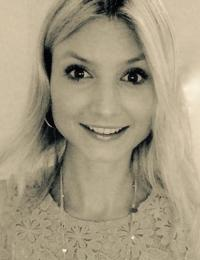Exponential-Type Time Integrators for Nonlinear Schrödinger, Korteweg-De Vries and Klein-Gordon Type Equations
Speaker
Prof. Katharina Schratz
Karlsruhe Institute of Technology, Germany
Abstract

In the context of the numerical time integration of (non)linear partial differential equations splitting methods as well as exponential integrators contribute attractive classes of integration methods. In recent years, they have in particular gained a lot of attention in the context of the numerical time integration of non-linear Schrödinger equations, the Korteweg-de Vries equation, semi-linear wave and Klein-Gordon type equations. In this talk I will present some recent developments in the construction of low-regularity exponential-type integrators for non-linear Schrödinger (NLS) equations as well as the Korteweg-de Vries (KdV) equation. The idea is thereby based on the discretization of Duhamel’s formula looking at the so-called “twisted variable” (in physics: interaction picture) which is obtained by a rescaling transformation with respect to the leading differential operator. This idea of “twisting the variable” is widely used in the analysis of partial differential equations in low regularity spaces and also well known in numerical analysis in the context of Lawson-type Runge-Kutta methods. However, instead of approximating the appearing integrals with a classical Runge-Kutta method we integrate, based on the Fourier decomposition of the exact solution, the dominant stiff parts exactly. This allows us to derive a new class of explicit exponential-type time integration schemes which converge under weaker regularity assumptions than classical methods. I will also outline how similar ideas can be used in the construction of uniformly accurate integration schemes for Klein-Gordon type equations in highly-oscillatory non-relativistic limit regimes where the speed of light formally tends to infinity. Thereby, the newly derived exponential-type integrators in particular allow asymptotic converge to classical splitting schemes in the non-linear Schrödinger limit.